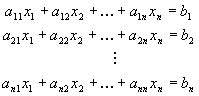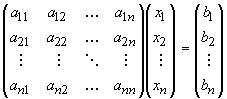SEARCH HOMEMath Central Quandaries & QueriesSubject: math Name: Avinash Who are you: Student Mary has 48 coins made up of nickels, dimes and quarters with a total value of $5.10. She has 4 more dimes than nickels and quarters combined. How many coins of each kind does she have? Use matrix to solve the system.Hi Avinash. I can get you started. Begin by assigning variable names to the unknowns: Let N = the number of nickels, D = the number of dimes, Q = the number of quarters. Then translate what you know into equations: "Mary has 48 coins made up of nickels, dimes and quarters" 48 = N + D + Q "with a total value of$5.10"
5.10 = 0.05N + 0.10D + 0.25Q

"She has 4 more dimes than nickels and quarters combined."
D = 4 + (N + Q)

This gives you three equations in three unknowns.  You are asked to
solve it using a matrix.  To do this, first re-arrange the three
equations into the form:The unknowns in this general form are denoted by x1, x2, ... xn and the
coefficients (a's and b's above) are the values given. In your case,
N, D and Q would be x1 through x3.

In matrix form the system of equations above can be written as:Write us back if you are uncertain about how to solve this matrix equation.
Stephen La Rocque.Math Central is supported by the University of Regina and The Pacific Institute for the Mathematical Sciences.